2x2-58x+396=0

Correct result:

x1 =  18
x2 =  11

#### Solution:

${x}_{1}=\frac{58+\sqrt{5{8}^{2}-4\cdot 2\cdot 396}}{2\cdot 2}=18$
${x}_{2}=\frac{58-\sqrt{5{8}^{2}-4\cdot 2\cdot 396}}{2\cdot 2}=11$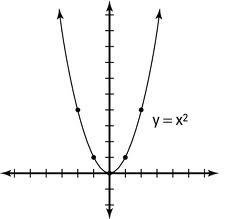We would be pleased if you find an error in the word problem, spelling mistakes, or inaccuracies and send it to us. Thank you!Tips to related online calculators

## Next similar math problems:

• Non linear eqsSolve the system of non-linear equations: 3x2-3x-y=-2 -6x2-x-y=-7
• Square root 2If the square root of 3m2 +22 and -x = 0, and x=7, what is m?
• Exponential equationSolve for x: (4^x):0,5=2/64.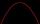Solve pure quadratic equation ?.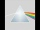Please determine the solvability conditions of the equation, solve the equation and perform the test: x divided by x squared minus 2x plus1 the whole minus x + 3 divided by x squared minus 1 this is equal to 0: x/(x2-2x+1) - (x+3)/( x2-1) = 0
• Trapezoid 15Area of trapezoid is 266. What value is x if bases b1 is 2x-3, b2 is 2x+1 and height h is x+4
• VCP equationSolve the following equation with variations, combinations and permutations: 4 V(2,x)-3 C(2,x+ 1) - x P(2) = 0
• Solve equationsolve equation: ?
• Linear systemSolve a set of two equations of two unknowns: 1.5x+1.2y=0.6 0.8x-0.2y=2
• Completing squareSolve the quadratic equation: m2=4m+20 using completing the square method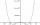By introducing a new variable solve biquadratic equation: ?Prove that k1 and k2 is the equations of two circles. Find the equation of the line that passes through the centers of these circles. k1: x2+y2+2x+4y+1=0 k2: x2+y2-8x+6y+9=0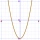F(x)=x2-7x and g(x)=5-x2 Domain of (fg)(x) is. .. . . Domain of (f/g)(x). ..Ellipse is expressed by equation 9x2 + 25y2 - 54x - 100y - 44 = 0. Find the length of primary and secondary axes, eccentricity, and coordinates of the center of the ellipse.Find the product and the sum of the roots of x2 + 3x - 9 = 0Find the roots of the quadratic equation: 3x2-4x + (-4) = 0.Solve quadratic equation: ?